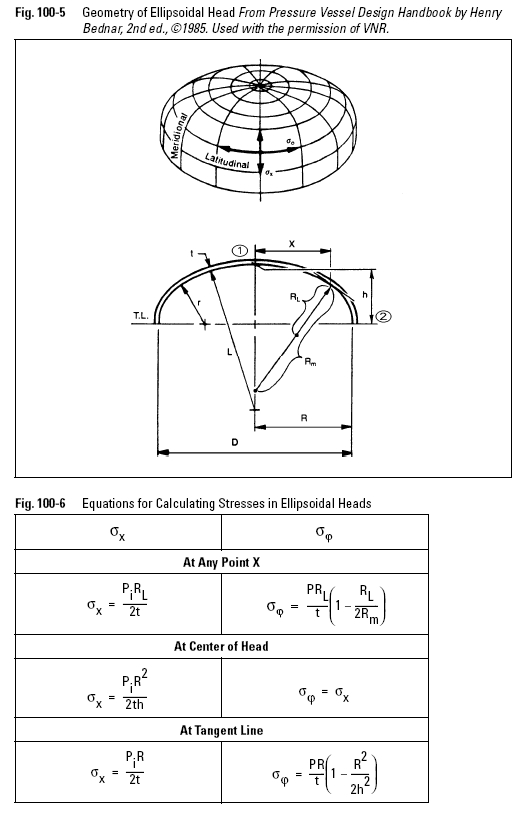﻿ Pressure Vessel Ellipsoidal Heads Under Internal Pressure – Oil & Gas Industry Technology Updates

## Pressure Vessel Ellipsoidal Heads Under Internal Pressure

Ellipsoidal heads, shown in Figure 100-5, are frequently used for the end closure of cylindrical shells. Since the radius of curvature varies from point to point, the equations for calculating the stress are more complicated than for cylinders or spheres. Both the meridional radius of curvature (RM) and the longitudinal radius of curvature (RL) vary gradually from point to point on the ellipse and therefore so do the meridional stress (Qx) and the latitudinal stress (Qo).

Figure 100-6 gives the equations for calculating the stresses in an ellipsoidal head. The meridional stress (Qx) remains tensile throughout the ellipsoid for all R/h ratios, being maximum at the crown (point 1) and diminishing in value to a minimum at the equator (point 2) (see Figure 100-5).

The latitudinal hoop stress (Qo) is also tensile in the crown region, but decreases toward the equator. For ratios R/h greater than 1.42, the hoop stress in the equator area becomes compressive. If R/h = 2, a maximum tensile meridional stress of Ph/t occurs at point 1, and a compressive latitudinal stress of equal magnitude occurs at
point 2. As R/h increases further, the greatest stress in the crown will still be tension at point 1, but will be far exceeded in magnitude by the compressive stress in the knuckle area at the equator. This is a potentially dangerous situation because this compressible stress can cause local buckling of thin heads and local failure due to the high shear stress developed. For this reason, the ASME Code restricts the major-to-minor-axis ratio of elliptical heads to a maximum of 2.

Using membrane stress alone for the design, without including the various effects of the discontinuity stresses at the head-to-shell junction, would result in insufficient head thickness. To simplify the design procedure, the ASME Code relates the equation for the thickness of ellipsoidal heads to the tangential stress of a cylindrical shell of radius R (the major radius of the ellipse), modified by an empirical corrective stress-intensification factor, “K.”